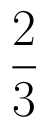Select Page

# 12 Science Maths CBSE Answers for MCQ Matrices in English

12 Science Maths CBSE Answers for MCQ Matrices in English to enable students to get Answers in a narrative video format for the specific question.

Expert Teacher provides 12 Science Maths CBSE Answers for MCQ Matrices through Video Answers in English language. This video solution will be useful for students to understand how to write an answer in exam in order to score more marks. This teacher uses a narrative style for a question from Matrices not only to explain the proper method of answering question, but deriving right answer too.

Please find the question below and view the Answer in a narrative video format.

Question:

## Similar Questions from CBSE, 12th Science, Maths, Matrices

Question 1 : Find the value of X, ifand. (View Answer Video)

Question 2 : If a matrix has 24 elements, what are the possible orders it can have? (View Answer Video)

Question 3 : Find the value of z,  from the equation:(View Answer Video)

Question 4 :  Find the value of z, if(View Answer Video)

Question 5 : Find the value of y from the equation:(View Answer Video)

### Application of Derivatives

Question 1 : For the curve, y=if x increases at the rate of 2 units/sec, then how fast is the slope of curve changing when x=3? (View Answer Video)

Question 2 : The point on the curvewhich is nearest to the point (0, 5) is  (View Answer Video)

Question 3 : Find the equation of the tangent to the curveat the points, where it cuts the x axis. (View Answer Video)

Question 4 : If, then the approximate value of f (3.02) is  (View Answer Video)

Question 5 : The approximate change in the volume of a cube of side x meters caused by increasing the side by 3% is _____________. (View Answer Video)

### Probability

Question 1 : A bag contains 4 red and 4 black balls, another bag contains 2 red and 6 black balls. One of the two bags is selected at random and two balls are drawn at random (without replacement) from the bag which are both found to be red. Find the probability that the balls are drawn from the first bag.    (View Answer Video)

Question 2 : A problem in mathematics is given to 4 students A, B, C, D. Their chances of solving the problem, respectively, areand. What is the probability that atmost one of them solve the problem?  (View Answer Video)

Question 3 :  A coin is tossed three times. Find P(F/E), where E : at most two tails and F : atleast one tail.  (View Answer Video)

Question 4 :  A die is rolled. If E= (1, 3, 5), F(2, 3) and G= {2, 3, 4, 5}, find(View Answer Video)

Question 5 : A bag contains (2n+1) coins. It is known that (n-1) of these coins have a head on both sides, whereas the rest of the coins are fair. A coin is picked up at random from the bag and is tossed. If the probability that the toss results in a head is, determine the value of n. (View Answer Video)

### Relations and Functions

Question 1 : Let R be the relation on the set N given by R ={(a, b): a = b - 2, b > 6}. Choose the correct answer. (View Answer Video)

Question 2 : Functionsare defined respectively, by, find. (View Answer Video)

Question 3 : Let A = {1, 2, 3}. Then, number of relations containing (1, 2) and (1, 3) which are reflexive and symmetric but not transitive is, (View Answer Video)

Question 4 : The number of binary operations which can be defined on the setis, (View Answer Video)